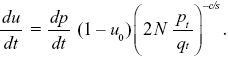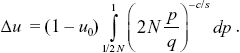NOTE 19DD: The exact result can be found using the methods of Box 28.2. We have du/dt = (dp/dt)(1 – u0) exp(–ct). Now, we know that pt/qt = (1/2N) exp(st), and so exp(–ct) = (2N pt/qt)c/s, andTreating u as a function of p (as in Box 28.2), we haveThis does not have an explicit solution, but for 2N large is very close to (2N)c/s, as given by the simple argument above.